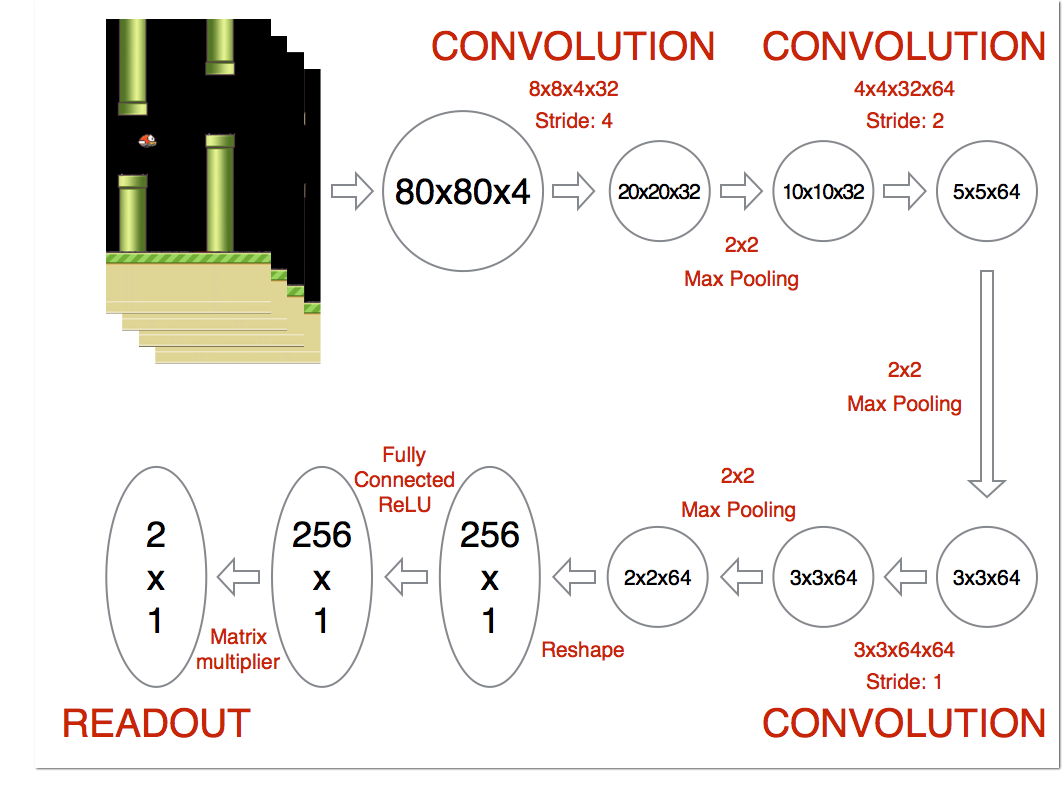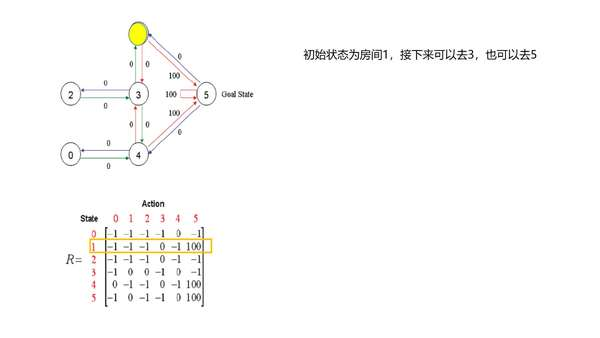## 1 学习目标

1. 复习Q-Learning；

2. 理解什么是值函数近似（Function Approximation）；

3. 理解什么是DQN，弄清它和Q-Learning的区别是什么。

## 2 用Q-Learning解决经典迷宫问题

Q-Learning是一种off-policy TD方法，伪代码如图所示https://www.zhihu.com/video/970368234306883584

Python代码：

import numpy as np
GAMMA = 0.8
Q = np.zeros((6,6))
R=np.asarray([[-1,-1,-1,-1,0,-1],
[-1,-1,-1,0,-1,100],
[-1,-1,-1,0,-1,-1],
[-1,0, 0, -1,0,-1],
[0,-1,-1,0,-1,100],
[-1,0,-1,-1,0,100]])
def getMaxQ(state):
return max(Q[state, :])
def QLearning(state):
curAction = None
for action in range(6):
if(R[state][action] == -1):
Q[state, action]=0
else:
curAction = action
Q[state,action]=R[state][action]+GAMMA * getMaxQ(curAction)
count=0
while count<1000:
for i in range(6):
QLearning(i)
count+=1
print(Q/5)

Q-Learning方法很好的解决了这个迷宫问题，但是这终究只是一个小问题（状态空间和动作空间都很小），实际情况下，大部分问题都是有巨大的状态空间或者动作空间，想建立一个Q表，内存是绝对不允许的，而且数据量和时间开销也是个问题。

## 3 值函数近似与DQN## 参考

Reinforcement Learning: An Introduction - Chapter 9: On-policy Prediction with Approximation

Reinforcement Learning: An Introduction - Chapter 10: On-policy Control with Approximation

David Silver's RL Course Lecture 6 - Value Function Approximation (video, slides)